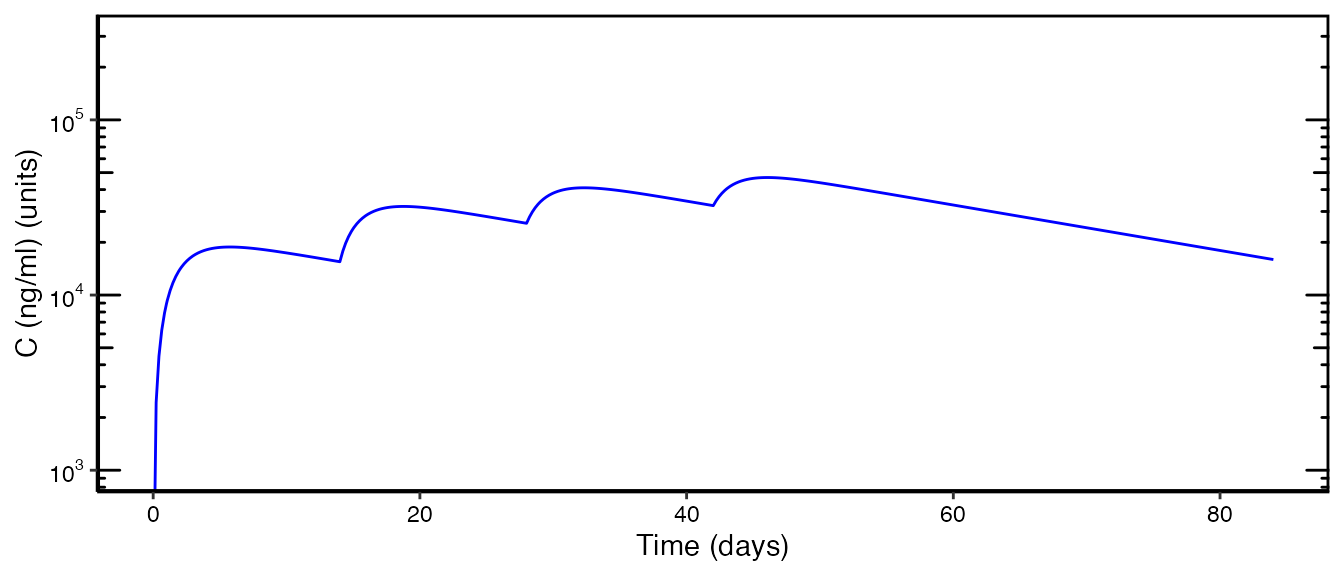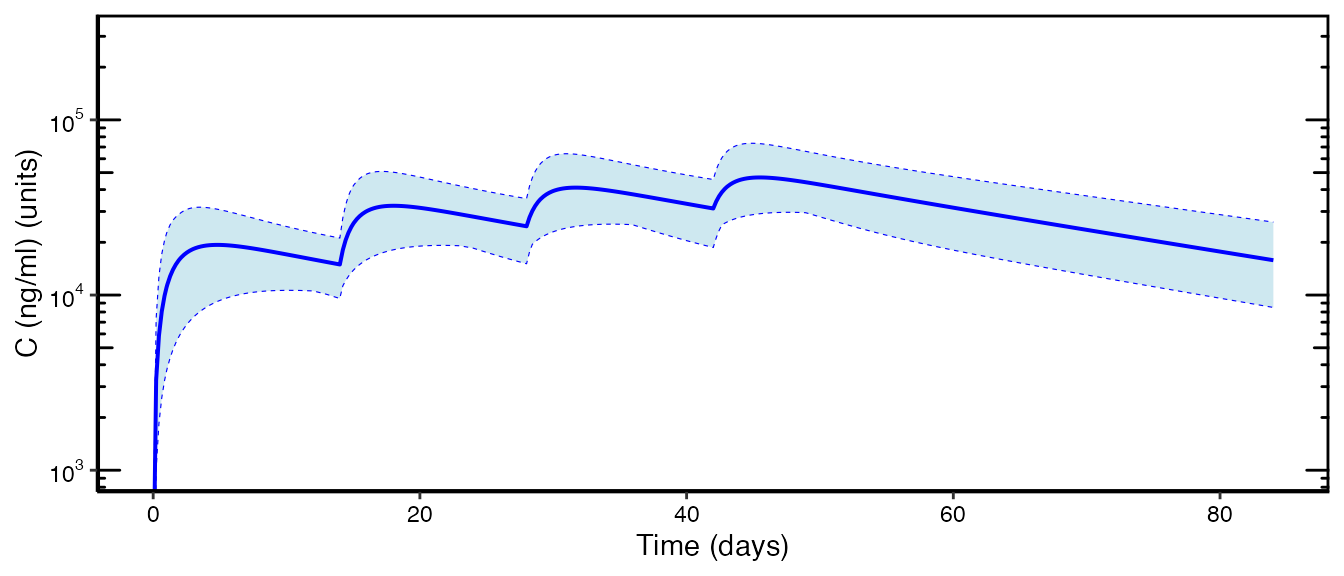## IntroductionThe workshop (workshop.ubiquity.tools) provides several examples of how to perform simulations in ubiquity. To make a copy of these scripts and other supporting files in the current working directory run the following:

library(ubiquity)
fr = workshop_fetch(section="Simulation", overwrite=TRUE)

This should create the following scripts:

• analysis_single.r Simulating the response for a single indiviudal
• analysis_multiple.r Mote Carlo simulations using IIV
• analysis_multiple_file.r Monte Carlo simulations reading subject information from a file

These rely on a PK model of mAbs in humans (Davda etal. mAbs, 6(4), 1094-1102). The contents of the system file for this model can be seen at the bottom (use ?system_new to see a list of the available system file examples). The first step in any analysis (simulation, estimation, etc) is building the system file creating the ubiquity model object (cfg).

cfg = build_system(system_file = "system.txt")

After building any system you can then create templates. To create a template script for running simulations use system_fetch_template and specify "Simulation" as the template argument:

system_fetch_template(cfg, template="Simulation")

This will create the file analysis_simulate.R in the working directory. This template should have common options and dosing information commented out. You simply need to uncomment them and run the simulation.

## Simulating an Individual Response (analysis_single.r)

We’ll begin by demonstrating how to simulate an indiviudal response to dosing. The system parameters for the default parameter set can be pulled out of this object:

cfg = build_system(system_file = "system.txt")
parameters = system_fetch_parameters(cfg)

To alter system parameters at the scripting level, you can just reassign the elements in parameters. For example to change the celarance to a value of .015 you could simply use: parameters$CL = 0.15. Next different simulation options can be set. For example the following will set the duration of the simulation to three months $$\left(3\ \mbox{months} \times 4\frac{\mbox{weeks}}{\mbox{month}}\times7\frac{\mbox{days}}{\mbox{week}}\right)$$ in days: cfg = system_set_option(cfg, group = "simulation", option = "output_times", seq(0,3*4*7,1)) The system file is written to allow both IV (Cp) and SC (At) dosing. So we zero out any default dosing specified in the system file. The next line specifies the SC dosing we want to simulate. cfg = system_zero_inputs(cfg) cfg = system_set_bolus(cfg, state = "At", times = c( 0, 14, 28, 42 ), # day values = c(200, 200, 200, 200 )) # mg Next we run the simulation: som = run_simulation_ubiquity(parameters, cfg) The variable som is a list containing the mapped simulation output, and the time course is stored in the simout element. The first column (time) contains the simulation time in the units of the simulation, days in this case. Next there is a column for each state (At, Cc, Cp) and a column for each output (C_ng_ml, C_DOSE). Each system parameter is passed through the simulation into the output (F1, … MW). This model has two covariates specified DOSE and WT. The initial value of these covariates is passed through as well as the values at each time point. For the covariate DOSE this is SIMINT_CVIC_DOSE and DOSE, respectively. Next secondary parameters are also provided (kel, … kpc). Lastly each timescale specified in the system file is also passed through with a “ts.” prefix: p = ggplot() + geom_line(data=som$simout, aes(x=ts.days, y=C_ng_ml), color="blue")  +
xlab("Time (days)")+
ylab("C (ng/ml) (units)")
p = gg_log10_yaxis(p, ylim_min=1e3, ylim_max=3e5)
p = prepare_figure("print", p)
print(p)## Simulating Population Response From IIV (analysis_multiple.r)

Next we want to simulate the response of multiple subjects. This system has IIV specified in the following manner:

 IIV details
IIV/Parameter set:
Short Name:  default
Variance/covariance matrix

ETAka             ETACL             ETAVc             ETAVp              ETAQ
ETAka            0.4160                 0                 0                 0                 0
ETACL                 0            0.0988            0.0786            0.0619                 0
ETAVc                 0            0.0786            0.1160            0.0377                 0
ETAVp                 0            0.0619            0.0377            0.0789                 0
ETAQ                 0                 0                 0                 0            0.6990

On parameters
Vp,      ETAVp(LN)
CL,      ETACL(LN)
Q,       ETAQ(LN)
ka,      ETAka(LN)
Vc,      ETAVc(LN)

Different aspects of the Monte Carlo simulations can be specified. For example the following states that we want to simulate 20 subjects and the simulation is run using simulate_subjects:

cfg=system_set_option(cfg, group  = "stochastic",
option = "nsub",
value  = 20)

som  = simulate_subjects(parameters, cfg)

The output here, som, has a different structure than the output from an individual simulation. It is a list with the following elements

• som$subjects$parameters - Matrix with a row for each subject containing that subjects parameters
• som$subjects$secondary_parameters - Matrix containing the static secondary parameters (one row for each subject)
• som$tcsummary - Data frame containing time course summary level information for the simulations there are columns for • Timescales (prefix ts.) • States (prefix s.) and Outputs (prefix o.) with the mean (suffix .mean) , median (suffix .median) and upper (suffix .ub_ci) and lower (suffix .lb_ci) bounds on the specified confidence interval (default 95%) • som$states - List with an element for each state. Each of these is a matrix containing the subject level predictions for that state (one row for each subject)
• som$output - List with an element for each output. Each of these is a matrix containing the subject level predictions for that output (one row for each subject) • som$times - Data frame with a column for the simulation time (time) and a column for each timescale with a ts. prefix.
p = ggplot(som\$tcsummary, aes(x=ts.days, y=o.C_ng_ml.mean)) +
geom_ribbon(aes(ymin=o.C_ng_ml.lb_ci,
ymax=o.C_ng_ml.ub_ci),
fill="lightblue",
alpha=0.6) +
geom_line(linetype="solid", size=0.7, color="blue")  +
geom_line(aes(x=ts.days, y=o.C_ng_ml.ub_ci), linetype="dashed", size=0.2, color="blue")  +
geom_line(aes(x=ts.days, y=o.C_ng_ml.lb_ci), linetype="dashed", size=0.2, color="blue")  +
xlab("Time (days)")+
ylab("C (ng/ml) (units)")+
guides(fill="none")

p     = gg_log10_yaxis(p    , ylim_min=1e3, ylim_max=3e5)
p     = prepare_figure("print", p    )
print(p)## Simulating Population Response From File (analysis_multiple_file.r)

Subject information can be pulled from a data file. First we need to load the dataset using system_load_data, here the dataset is named SUBS.

cfg = system_load_data(cfg,
dsname    = "SUBS",
data_file = system.file("ubinc", "csv", "mab_pk_subjects.csv",
package = "ubiquity"))

The format of the dataset is shown below. There needs to be an column for the subject ID (SIMINT_ID) and the simulation time (SIMINT_TIME). Next the subject level parameters can be specified where the column headers correspond to the parameter names. If a parameter is not specified, the default value will be taken from the parameters input. In this case MW was not specified in the dataset, so it will be taken from parameters.

Optionally, covariates can also be specified by name as well. For time varying covariates, multiple records can be specified for the same subject (the parameter values for that subject should remain constant between records). In this example WT and SEX are covariates but only WT has been defined in the system file, so SEX will be ignored. The covariate DOSE is not defined so the default from the system file will be used.

With the dataset loaded, we need to link that subject file to the stochastic simulations using the dataset name (SUBS) and then we can tell the scripts how to handle sampling from the dataset:

cfg=system_set_option(cfg, group  = "stochastic",
option = "sub_file",
value  = "SUBS")

cfg=system_set_option(cfg, group  = "stochastic",
option = "sub_file_sample",
value  = "with replacement")

Then we just use simulate_subjects to run the simulation as before:

som  = simulate_subjects(parameters, cfg)

## Parallelization

If you are using simulate_subjects to perform population simulations and have multiple cores on your computer you can utilize those cores by setting the following options in the "simulation" group:

cfg=system_set_option(cfg, group  = "simulation",
option = "parallel",
value  = "multicore")

cfg=system_set_option(cfg, group  = "simulation",
option = "compute_cores",
value  = detectCores() - 1)

## Contents of system.txt

# Implementation of the two compartment model from Davda 2014
#
#   Davda, J. P., Dodds, M. G., Gibbs, M. A., Wisdom, W., & Gibbs, J. (2014). A
#   model-based meta-analysis of monoclonal antibody pharmacokinetics to guide
#   optimal first-in-human study design. mAbs, 6(4), 1094-1102.
#   http://doi.org/10.4161/mabs.29095
#
# System Units:
#   mass          [=] nmoles
#   volume        [=] L
#   concentration [=] nM
#   time          [=] day
#

# #-------------#
# | Parameters  |
# #-------------#
# System parameters
#name          value          lower  upper    units  editable grouping
#                             bound  bound
<P> F1         0.744          eps    inf      ---    yes      System
<P> ka         0.282          eps    inf      1/day  yes      System
<P> CL         0.200          eps    inf      L/day  yes      System
<P> Vc         3.61           eps    inf      L      yes      System
<P> Vp         2.75           eps    inf      L      yes      System
<P> Q          0.747          eps    inf      L/day  yes      System
<P> MW         140            eps    inf      kD     yes      System
<PSET:default>  mAb in Humans

# Interindividual Variability
# Taken from Table 3
<IIV:ETAka>    0.416
<IIV:ETAka:LN> ka

<IIV:ETACL>    0.09875
<IIV:ETACL:LN> CL

<IIV:ETAVc>    0.116
<IIV:ETAVc:LN> Vc

<IIV:ETAVp>    0.0789
<IIV:ETAVp:LN> Vp

<IIV:ETAQ>     0.699
<IIV:ETAQ:LN>  Q

<IIVCOR:ETACL:ETAVc>   0.0786
<IIVCOR:ETACL:ETAVp>   0.0619
<IIVCOR:ETAVp:ETAVc>   0.0377

# Covariates
<CV:DOSE>    ; times;      [ 0 ];    day
<CV:DOSE>    ; values;     ;    mg
<CVTYPE:DOSE> step

<CV:WT>      ; times;      [ 0 ];    day
<CV:WT>      ; values;     [ 60];    kg
<CVTYPE:WT>   step

# static secondary parameters
<As> kel = CL/Vc
<As> kcp = Q/Vc
<As> kpc = Q/Vp

# #-------------------#
# |Input Information |
# #-------------------#
#
#        1e6 ng    1 nmole             1
# X mg x ------ x ----------------- x ---  =>  X*1e3/MW/Vc
#        1 mg      MW (KDA) * 1000    V(L)
#
# Bolus Events
# times/events state   values              scale    units
<B:times>;           [  0.0, 7, 14 ];          1;   days
<B:events>;   At;    [400.0, 0, 0  ];     1e3/MW;   mg
<B:events>;   Cc;    [  0.0, 0, 0  ];  1e3/MW/Vc;   mg

<R:Dinf>;    times;     [0, 30];     1/60/24;           min
<R:Dinf>;    levels;    [0,  0];       60*24*1e3/MW;    mg/min

# ODEs
<ODE:At> -ka*At
<ODE:Cc>  ka*At*F1/Vc  -kel*Cc - kcp*Cc  + kpc*Cp*Vp/Vc + Dinf/Vc
<ODE:Cp>                                 + kcp*Cc*Vc/Vp - kpc*Cp

# #---------#
# | Outputs |
# #---------#
# Outputs that begin with QC will not be displayed in the GUI

# Convert nM to ng/ml
#
#  X nM  ===> X*MW(KDA)
#
# Convert nM to ug/ml/mg(dose)
#
#  X nM  ===> X*MW(KDA)/1000/dose
#
<O> C_ng_ml = Cc*MW
<O> C_DOSE  = Cc*MW/DOSE/1000

# #---------#
# | Options #
# #---------#
# General Options:
# specify different time scales
<TS:min>   24.0*60.0
<TS:days>  1.0
<TS:hours> 24.0
<TS:weeks> 1.0/7.0
<TS:months> 1.0/7.0/4.0